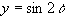I. THE PYTHAGORAS' THEORM

1. Simplify the following:

(a) sin7x.cos6x - cos7x.sin6x (b) 2sin3q.cos3q

(c)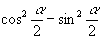(d)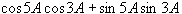(e)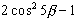(f)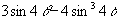(g)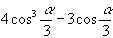(h)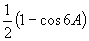(i)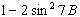(j)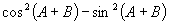2. Simplify the following:

(a)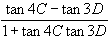(b)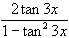(c)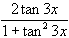(d)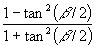(e)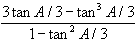(f)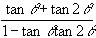3. Evaluate the following:

(a)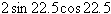(b)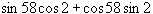(c)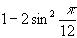(d)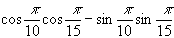(e)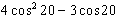(f)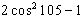(g)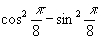(h)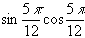4. Find the exact value of the following:

(a)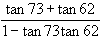(b)(c)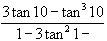(d)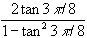(e)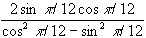(f)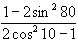5. If A and B are acute and sinA = 4/5 and cosB=12/13, determine the values of :

(a) cosA (b) sin2A (c) sin(A-B)

6. If x is acute and cosx = 1/3, find the exact values of:

(a) cos(2x) (b) cos(3x) (c) tan(x/2)

7. If tan(x) = 1/2 and x+y = p/4, find the values of :

(a) tan(2x) (b) tan(3x) (c) tan(y)

8. Write down the result of cos(x + y) hence

(a) simplify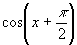(b) show that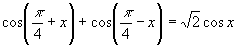(c) prove that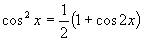9. (i) Assuming results for cos(a + b), cosq, sin2q , find an expression for cos3b in terms of cosb.

If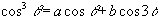for all values of q, find the values of the constants a, b.

(ii) Write down the result for tan2q in terms of tanq . If tan2q = 5/12, where 0<2q<p/2, find, the value of tanq.

10. (i) Write down the result for tan(a - b) and hence show that tan15 = 2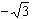(ii) Show that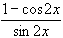= tanx , and use this result to obtain tan(p/12) in simplest form.

11. (i) Prove that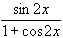= tanx and hence find the value of tan(p/8) in simplest surd form.

(ii) Show that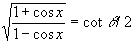, where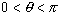, and prove that cos67.5=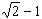.

12. Show that cos(x + y) + cos(x - y) = 2cosx.cosy and hence find an expression for

(a) cos75.cos35 in thr form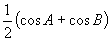(b) cos75 + cos35 in the form 2cosA.cosB

13. Find an expression for

(i) sin25.cos10 in the form(ii) cos60 - cos80 in the form 2cosA.cosB

14. (i) Write down an expression for sin(a + b) and hence show that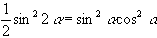If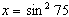, evaluate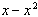(ii) Prove that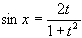where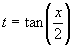, and hence show that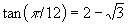.

15. (i) Starting with the result for cos(x + y), show that 1 - cos2x = 2.sin2x

(ii) Determine the following limits as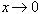(a)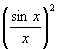(b)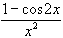(c)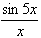(d)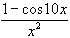.

16. Use the result of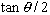to prove that

(a)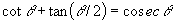(b)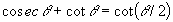17. Prove the following identities:

(a)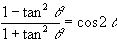(b)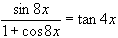(c)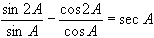(d)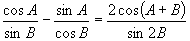18. Show that

(a)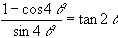(b)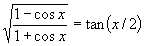(c)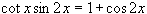(d)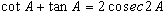19. Prove each of the following:

(a)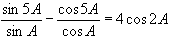(b)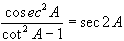(c)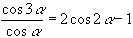(d)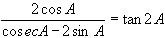20. Show that

(a)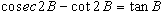(b)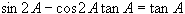(c)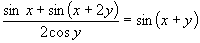(d)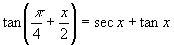21. (i) Show that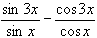is independent of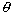. What is its value ?

(ii) Ifis acute and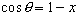, where x is so small that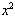is negligible compared with unity, prove that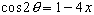22. (i) If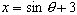,, show that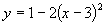(ii) If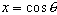and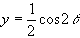, find an equation linking x, y which is independent of.

(iii) Eliminatein each of the following:

(a)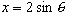,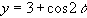(b)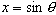,